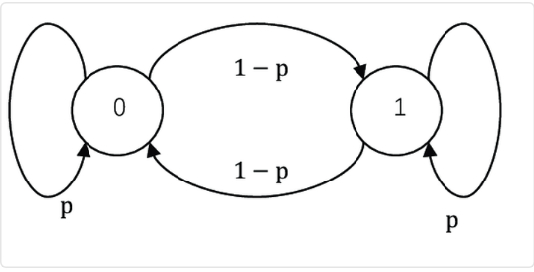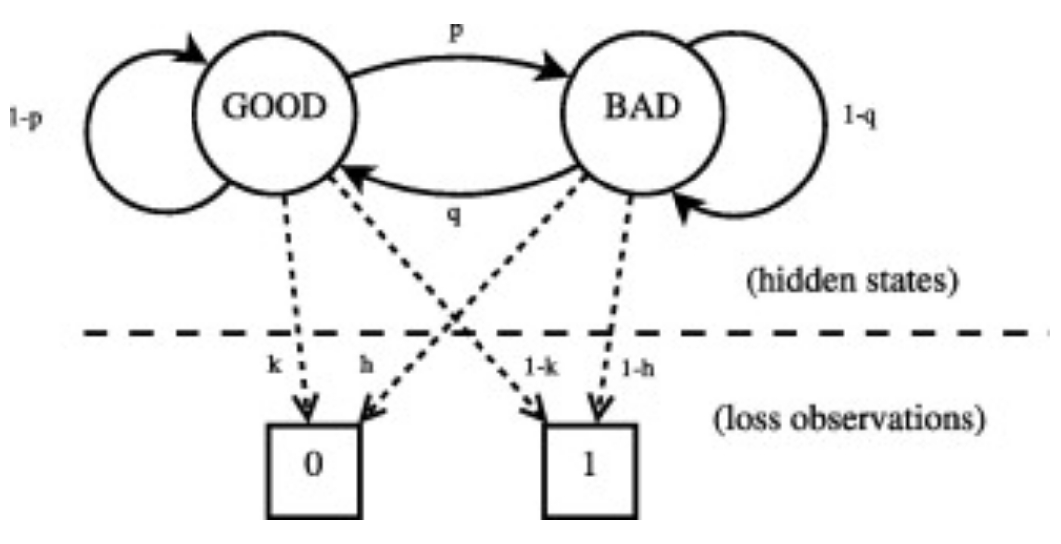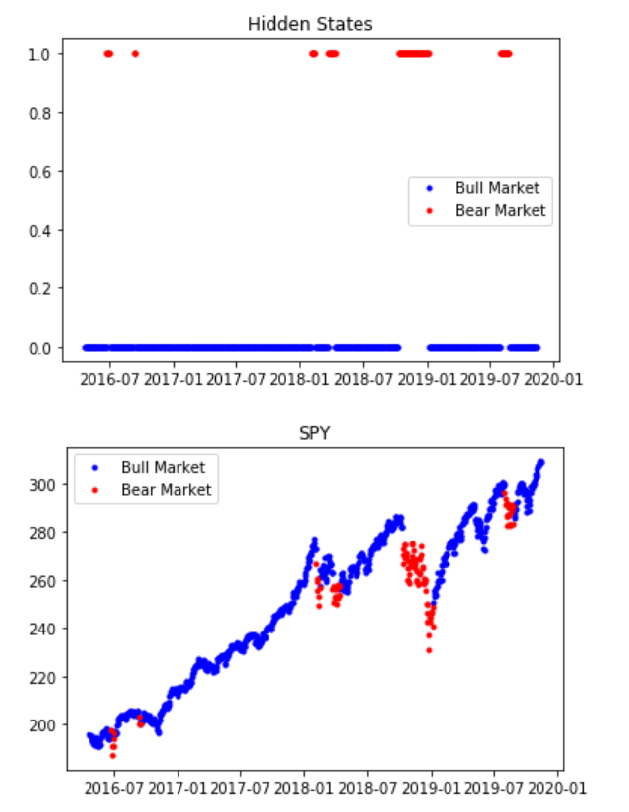One of the most important aspects of trading is risk management. Knowing when the market is going to move against you and acting before this happens is the best way to avoid large drawdowns and maximize your Sharpe ratio. The ultimate strategy is buy-low, sell-high. The trouble with this, however, is knowing when low is low and high is high. Hindsight is 20/20, but can we find a way to understand when markets are about to shift from bull to bear?

One possibility is to use a Hidden Markov Model (HMM). These are Markov models where the system is being modeled as a Markov process but whose states are unobserved, or hidden. (Briefly, a Markov process is a stochastic process where the possibility of switching to another state depends only on the current state of the model -- it is history-independent, or memoryless). In a regular Markov model, the state is observable by the user and so the only parameters are the state transition probabilities. For example, in a two-state Markov model, the user is able to know which state the system being modeled is in, and so the only model parameters to be characterized are the probabilities of attaining each state.In an HMM, each state has transition probabilities and there are underlying latent states, but they are not directly observable. Instead, they influence the observations which are the only observable element.As with Kalman filters, there are three main aspects of interest to us:

1. Prediction - forecasting future values of the process
2. Filtering - estimating the current state of the model
3. Smoothing - estimating past states of the model

The salient feature here is prediction as our ultimate goal is to predict the market state. To experiment with this, we used the research notebook to get historical data for SPY and fit a Gaussian, two-state Hidden Markov Model to the data. We built a few functions to build, fit, and predict from our Gaussian HMM.

```from HMM import * import numpy as np from scipy.stats import jarque_bera symbol = qb.AddEquity('SPY', Resolution.Daily).Symbol # Fetch history and returns history = qb.History(symbol, 500, Resolution.Hour) returns = history.close.pct_change().dropna() # Define Hidden Markov Model functions def CreateHMM(algorithm, symbol): history = algorithm.History([symbol], 900, Resolution.Daily) returns = np.array(history.loc[symbol].close.pct_change().dropna()) # Reshape returns returns = np.array(returns).reshape((len(returns),1)) # Initialize Gaussian Hidden Markov Model model = GaussianHMM(n_components=2, covariance_type="full", n_iter=1000).fit(returns) print(model.score(returns)) return model def PlotStates(algorithm, symbol, model): history = algorithm.History([symbol], 900, Resolution.Daily).loc[symbol] returns = history.close.pct_change().dropna() hidden_states = model.predict(np.array(returns).reshape((len(returns),1))) hidden_states = pd.Series(hidden_states, index = returns.index) hidden_states.name = 'hidden' bull = hidden_states.loc[hidden_states.values == 0] bear = hidden_states.loc[hidden_states.values == 1] plt.figure() ax = plt.gca() ax.plot(bull.index, bull.values, ".", linestyle='none', c = 'b', label = "Bull Market") ax.plot(bear.index, bear.values, ".", linestyle='none', c = 'r', label = "Bear Market") plt.title('Hidden States') ax.legend() plt.show() df = history.join(hidden_states, how = 'inner') df = df[['close', 'hidden']] up = pd.Series() down = pd.Series() mid = pd.Series() for tuple in df.itertuples(): if tuple.hidden == 0: x = pd.Series(tuple.close, index = [tuple.Index]) up = up.append(x) else: x = pd.Series(tuple.close, index = [tuple.Index]) down = down.append(x) up = up.sort_index() down = down.sort_index() plt.figure() ax = plt.gca() ax.plot(up.index, up.values, ".", linestyle='none', c = 'b', label = "Bull Market") ax.plot(down.index, down.values, ".", linestyle='none', c = 'r', label = "Bear Market") plt.title('SPY') ax.legend() plt.show() # Build the model and plot good/bad regime states model = CreateHMM(qb, symbol) PlotStates(qb, symbol, model)```As we can see, the HMM we fit with SPY returns data does a reasonably good job of detecting bear markets bull markets. To apply this in a model, we decided to use it as a way to manage risk in the algorithm developed using stationarity and z-scores. The basic mean-reversion strategy remains, but instead, we add the condition to enter a position that the security must be in a bull-state, and all positions are exited and no trading happens during a bear-state.

(The specific application of an HMM to SPY returns and as use in risk management is thanks in part to articles on HMM and trading found here and here.)

To do this, we initialize and fit a Gaussian, two-state HMM model for each security when they are added to the universe.

``` def OnSecuritiesChanged(self, changes): symbols = [x.Symbol for x in changes.AddedSecurities] # Build model for each symbol for symbol in symbols: self.models[symbol] = CreateHMM(self, symbol)```

Every day, we run our tests for stationarity, get z-scores, make our state-prediction, and then iterate over all of the symbols and apply our trading logic.

``` def GenerateInsights(self): insights = [] qb = self symbols = [x.Symbol for x in qb.ActiveSecurities.Values] # Copy and paste from research notebook # ----------------------------------------------------------------------------- # Fetch history history = qb.History(symbols, 500, Resolution.Hour) # Convert to returns returns = history.unstack(level = 1).close.transpose().pct_change().dropna() # Test for stationarity stationarity = TestStationartiy(returns) # Get z-scores z_scores = GetZScores(returns) # ----------------------------------------------------------------------------- insights = [] # Iterate over symbols for symbol, value in stationarity.iteritems(): # Only emit Insights for those whose returns exhibit stationary behavior if value: # Get Hidden Markov model model = self.CheckForHMM(symbol) # Predict current state state_prediction = PredictState(self, model, symbol) # Get most recent z_score z_score = z_scores[symbol].tail(1).values # Determine if we want to invest or not if (z_score < -1) and (state_prediction == 0): insights.append(Insight.Price(symbol, timedelta(1), InsightDirection.Up)) elif z_score > 1: if self.Portfolio[symbol].Invested: insights.append(Insight.Price(symbol, timedelta(1), InsightDirection.Flat)) elif self.Portfolio[symbol].Invested and (state_prediction == 1): insights.append(Insight.Price(symbol, timedelta(1), InsightDirection.Flat)) self.EmitInsights(insights)```

Finally, we re-fit the models every 30 days.

``` def RefitModels(self): for symbol, model in self.models.items(): RefitModel(self, symbol, model)```

All of this is done using an Immediate Execution Model, Equal Weighting Portfolio Construction Model, and the Liquid ETF Universe.

``` def Initialize(self): self.SetStartDate(2019, 1, 1) # Set Start Date self.SetCash(100000) # Set Strategy Cash self.SetBrokerageModel(AlphaStreamsBrokerageModel()) self.SetExecution(ImmediateExecutionModel()) self.SetPortfolioConstruction(EqualWeightingPortfolioConstructionModel()) self.SetUniverseSelection(LiquidETFUniverse()) self.models = {} self.AddEquity('GLD') self.Schedule.On(self.DateRules.EveryDay('GLD'), self.TimeRules.AfterMarketOpen('GLD', 5), self.GenerateInsights) self.Schedule.On(self.DateRules.MonthStart('GLD'), self.TimeRules.At(19,0), self.RefitModels)```

As can be seen in the attached backtest, using Hidden Markov Models to detect optimal market conditions actually decreased our algorithm's performance vs the algorithm using just stationarity tests and z-scores, but this doesn't mean that we shouldn't continue to experiment with them. Perhaps a two-state HMM was great at predicting bear/bull states for index ETFs but not for sector ETFs and applying the way we did here was a mistake. Either way, detecting bear/bull market states are hardly the only application of Hidden Markov Models in finance. These can be used to detect when time-series attain various states with respect to all sorts of statistical properties, and their application can be in risk-management, portfolio construction, execution, or determining optimal trading rules.

Have fun and good luck!